So far you have learnt that square matrix has determinant value and larger the square matrix more difficult it is to compute the determinant through cross multiplication method. The larger matrix is usually broken down into smaller matrix and determinant or minors are calculated. Then assigning signs to these minors will give you cofactors to computer the determinant of the original matrix. Here we will discuss about computing adjoint of square matrix using cofactors.

The adjugate of a square matrix is also called adjoint matrix which is obtained from matrix of cofactors. To learn about cofactors visit : Cofactors of square matrix.

Suppose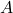is a square matrix , and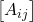is its cofactor matrix. Then the adjoint of the matrixcan be obtained by transposing the cofactor matrix.

Letbe a matrix of size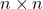,andis the cofactor matrix of. The adjoint of the matrixis denoted as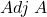.

Steps to find Adjoint of a Square Matrix

The steps to find the adjoint of a square matrix is easy as most of the steps you have learned in previous articles already. Here are the steps.

1. Check if the given matrixis asquare matrix.
2. Find the minors of the matrixand arrange them in a matrix form according to subscript value.
3. Find the cofactors of matrixfrom the given minor matrix and arrange in matrix form according to subscript of each cofactor.
4. Transpose the cofactor matrix to obtain the adjoint of matrixExample #1

Find the adjoint of matrix.

A = \begin{bmatrix}2 & 1 & 3\\1 & 5 & 2\\4 & 7 & 3\end{bmatrix}

Solution:

We will find solution to this matrix in a step-by-step manner.

Step1: The given matrixis a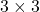square matrix.

A = \begin{bmatrix}2 & 1 & 3\\1 & 5 & 2\\4 & 7 & 3\end{bmatrix}

Step2: We must find the matrix of minors for.

\begin{aligned}
&M_{11} = \begin{vmatrix}5 & 2\\7 & 3\end{vmatrix} = 15-14 = 1\\\\
&M_{12} = \begin{vmatrix}1 & 2\\4 & 3\end{vmatrix} = 3-8 = -5\\\\
&M_{13} = \begin{vmatrix}1 & 5\\4 & 7\end{vmatrix} = 7-20 = -13\\\\
&M_{21} = \begin{vmatrix}1 & 3\\7 & 3\end{vmatrix} = 3-21 = -18\\\\
&M_{22} = \begin{vmatrix}2 & 3\\4 & 3\end{vmatrix} = 6-12 = -6\\\\
&M_{23} = \begin{vmatrix}2 & 1\\4 & 7\end{vmatrix} = 14-4 = 10\\\\
&M_{31} = \begin{vmatrix}1 & 3\\5 & 2\end{vmatrix} = 2-15 = -13\\\\
&M_{32} = \begin{vmatrix}2 & 3\\1 & 2\end{vmatrix} = 4-3 = 1\\\\
&M_{33} = \begin{vmatrix}2 & 1\\1 & 5\end{vmatrix} = 10-1 = 9\\\\
&= \begin{bmatrix}1 & -5 & -13\\-18 & -6 & 10\\-13 & 1 & 9\end{bmatrix}
\end{aligned}

Step3: Given the matrix of minors we can quickly find the cofactor matrix by shortcut method. Assign negative or positive sign to minors based on following pattern.

 = \begin{bmatrix}+ & - & +\\- & + & -\\+ & - & +\end{bmatrix}

Then we get,

\begin{aligned}
&= \begin{bmatrix}(+)1 & (-)-5 & (+)-13\\(-)-18 & (+)-6 & (-)10\\(+)-13 & (-)1 & (+)9\end{bmatrix}\\\\
&\begin{bmatrix}A_{ij}\end{bmatrix} = \begin{bmatrix}1 & 5 & -13\\18 & -6 & -10\\-13 & -1 & 9\end{bmatrix}
\end{aligned}

Step4: Finally, transpose the cofactor matrix to get the adjoint of matrix.

\begin{aligned}
&Adj A = \begin{bmatrix}A_{ij}\end{bmatrix}^T = \begin{bmatrix}1 & 5 & -13\\18 & -6 & -10\\-13 & -1 & 9\end{bmatrix}^T\\\\
&Adj A = \begin{bmatrix}1 & 18 & -13\\5 & -6 & -1\\-13 & -10 & 9\end{bmatrix}
\end{aligned}

The adjoint matrix can also be used to find the inverse of a matrix. The only condition is that the determinant must not be zero. If the determinant of a square matrix is nonzero, then the following will give inverse matrix of a matrix.

A^{-1} = \frac{1}{|A|}\times Adj A

In the previous example, we computed the adjoint matrix of A which is

Adj \hspace{3px}A = \begin{bmatrix}1 & 18 & -13\\5 & -6 & -1\\-13 & -10 & 9\end{bmatrix}

Let us find the determinant of the matrix by multiplying first row of matrix A and first row of cofactor matrix of A.

\begin{aligned}
&Row \hspace{3px}1 \hspace{3px}of \hspace{3px}A = 2 \hspace{6px}1 \hspace{6px}3 \\\\
&Row \hspace{3px}1\hspace{3px} of\hspace{3px} Cofactor\hspace{3px} of \hspace{3px}A = 1 5 -13
\end{aligned}

Therefore,the deterinant of matrix A is,

|A| = 2(1) + 1(5) + 3 (-13) = 2 + 5 -39 = -32

We can compute the inverse of matrixusing determinant and the adjoint matrix.

\begin{aligned}
&A^{-1} = \frac{1}{-32} \times \begin{bmatrix}1 & 18 & -13\\5 & -6 & -1\\-13 & -10 & 9\end{bmatrix}\\\\
&A^{-1} = \begin{bmatrix}-1/32& -9/16&13/32\\ -5/32&3/16&1/32\\13/32&5/16&-9/32\end{bmatrix}
\end{aligned}

Readers must check the correctness of the inverse as an exercise.

In the next article, we will discuss about how to find the solution to a system of linear equations using determinant method popularly known as Cramer’s rule.